# Engineering Mechanics - KOP: Work and Energy

### Exercise :: KOP: Work and Energy - General Questions

• KOP: Work and Energy - General Questions
6.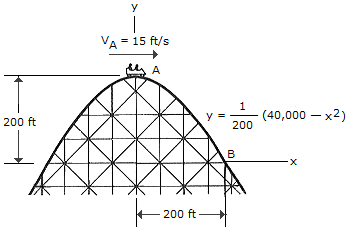The roller-coaster car has a speed of 15 ft/s when it is at the crest of a vertical parabolic track. Compute the velocity and the normal force it exerts on the track when it reaches point B. Neglect friction and the mass of the wheels. The total weight of the car and the passengers is 350 lb.

 A. vB = 114.5 ft/s, NB = 29.1 lb B. vB = 114.5 ft/s, NB = 284 lb C. vB = 114.5 ft/s, NB = 156.5 lb D. vB = 114.5 ft/s, NB = 440 lb

Explanation:

No answer description available for this question. Let us discuss.

7.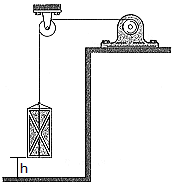A motor hoists a 50-kg crate at constant speed to a height of h = 6 m in 3 s. If the indicated power of the motor is 4 kw, determine the motor's efficiency.

 A. e = 0.025 (2.5%) B. e = 0.245 (24.5%) C. e = 0.736 (73.6%) D. e = 0.05 (5.0%)

Explanation:

No answer description available for this question. Let us discuss.

8.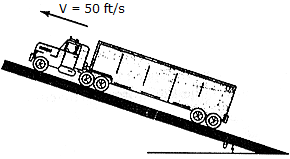A truck has a weight of 25,000 lb and an engine which transmits a power of 350hp. Assuming that the wheels do not slip on the ground, determine the angleof the largest incline the truck can climb at a constant speed of v = 50 ft/s.

 A. 2 = 8.86E B. 2 = 24.3E C. 2 = 8.75E D. 2 = 26.8E

Explanation:

No answer description available for this question. Let us discuss.

9.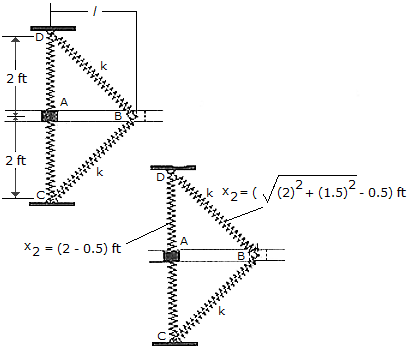The book A having a weight of 1.5 lb slides on the smooth horizontal slot. If the block is drawn back so that s = 0. Each of the two springs has a stiffness of k = 150 lb/ft and an unstretched length of 0.5 ft.

 A. vA = 106.2 ft/s B. vA = 120.4 ft/s C. vA = 160.5 ft/s D. vA = 107.7 ft/s

Explanation:

No answer description available for this question. Let us discuss.

10.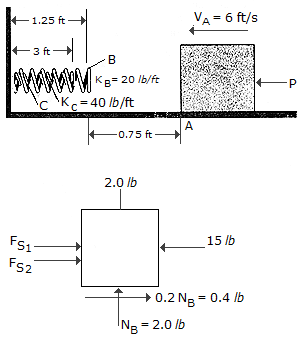The coefficient of friction between the 2-lb block and the surface is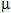= 0.2. The block is acted upon by a horizontal force of P. Determine the maximum deformation of the outer spring B at the instant the block comes to rest. Spring B has a stiffness of KB = 20 lb/ft and the "nested" spring C has a stiffness of kc = 40 lb/ft.

 A. xB = 1.154 ft B. xB = 0.790 ft C. xB = 0.923 ft D. xB = 1.137 ft